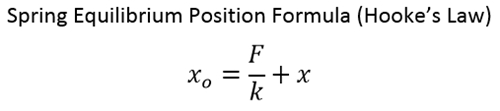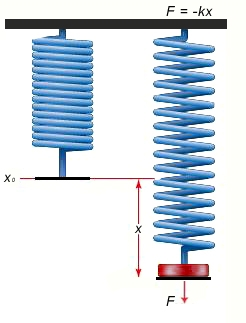# Spring Equilibrium Position Calculator

Newton/Meter
Newton
Meter

#### ResultClick here to view image

Where:

xo = The equilibrium position

x = The displacement of the spring from its equilibrium (Can be negative or positive, based on whether the spring is compressed or stretched)

k = Spring Constant

F = Force required to stretch the spring